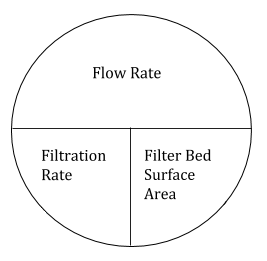# 6.2: Filtration Rates

$$\newcommand{\vecs}{\overset { \rightharpoonup} {\mathbf{#1}} }$$ $$\newcommand{\vecd}{\overset{-\!-\!\rightharpoonup}{\vphantom{a}\smash {#1}}}$$$$\newcommand{\id}{\mathrm{id}}$$ $$\newcommand{\Span}{\mathrm{span}}$$ $$\newcommand{\kernel}{\mathrm{null}\,}$$ $$\newcommand{\range}{\mathrm{range}\,}$$ $$\newcommand{\RealPart}{\mathrm{Re}}$$ $$\newcommand{\ImaginaryPart}{\mathrm{Im}}$$ $$\newcommand{\Argument}{\mathrm{Arg}}$$ $$\newcommand{\norm}{\| #1 \|}$$ $$\newcommand{\inner}{\langle #1, #2 \rangle}$$ $$\newcommand{\Span}{\mathrm{span}}$$ $$\newcommand{\id}{\mathrm{id}}$$ $$\newcommand{\Span}{\mathrm{span}}$$ $$\newcommand{\kernel}{\mathrm{null}\,}$$ $$\newcommand{\range}{\mathrm{range}\,}$$ $$\newcommand{\RealPart}{\mathrm{Re}}$$ $$\newcommand{\ImaginaryPart}{\mathrm{Im}}$$ $$\newcommand{\Argument}{\mathrm{Arg}}$$ $$\newcommand{\norm}{\| #1 \|}$$ $$\newcommand{\inner}{\langle #1, #2 \rangle}$$ $$\newcommand{\Span}{\mathrm{span}}$$$$\newcommand{\AA}{\unicode[.8,0]{x212B}}$$

One of the most important processes in a water treatment plant is filtration. It is the last barrier between the treatment process and the customer. Filters trap or remove particles from the water further reducing the cloudiness or turbidity. There are different shapes, sizes, and types of filters containing one bed or a combination of beds of sand, anthracite coal, or some other form of granular material.

Slow sand filters are the oldest type of municipal water filtration and have filtration rates varying from 0.015 to 0.15 gallons per minute per square foot of filter bed area, depending on the gradation of filter medium and raw water quality. Rapid sand filters, on the other hand, can have filtration rates ranging from 2.0 to 10 gallons per minute per square foot of filter bed area. Typically rapid sand filters will require more frequent backwash cycles to remove the trapped debris from the filters.

Backwashing is the reversal of flow through the filters at a higher rate to remove clogged particles from the filters. Backwash run times can be anywhere from 5–20 minutes with rates ranging from 8 to 25 gallons per minute per square foot of filter bed area, depending on the quality of the pre-filtered water.Figure $$\PageIndex{1}$$

Filtration and backwash rates are calculated by dividing the flow rate through the filter by the surface area of the filter bed. Typically these rates are measured in gallons per minute per square foot of filter bed area.

• Flow Rate (gpm)/Surface Area (sq.ft) = Filtration Rate

Although filtration rates are commonly expressed as gpm/ft2 they are also expressed as the distance of fall (in inches) within the filter per unit of time (in minutes). During backwashing it is expressed with the same units only per “rise” in the filter. See the example below.

• Filtration Rate = Fall (inches)/Time (min)
• Backwash Rate = Rise (inches)/Time (min)

## Examples

Express 2.5 gpm/ft2 as in/min.

First, convert gpm to cfm. The purpose of this is to begin matching the unit of “inches” in in/min to the “cubic feet in cfm.

• (2.5 gal/min)/sq.ft x 1 cf/7.48 gal = 0.33 cfm/sq.ft
• 2.5 gpm/sq.ft x 1 cf/7.48 gal = 0.33 cfm/sq.ft

If you look at the above answer of (0.33 cf/m)/sq.ft more closely you can see that it can also be expressed as 0.33 ft/min since the sq.ft and cf cancel each other to feet.

Once you have “feet per min” you can easily convert to “inches per min” by multiplying by 12.

• 0.33 ft/min x 12 in/1 ft = 4 in/min

Let’s try another example: What is the filtration rate through a 20’ by 20’ filter if the average flow through the treatment is 2.5 MG?

First, convert 2.5 MGD to gpm. To do this divide 2.5 MGD by 1,440.

• 2,500,000 gal/day x 1 day/1,440 min = 1,736 gpm

Then divide the flow by the area of the filter (20’ x 20’ = 400 sq.ft).Figure $$\PageIndex{2}$$
• 1,736 gpm/400 sq.ft = 4.34 gpm/sq.ft

Now, calculate the inches per minute.

Instead of 7.48 gallons per cubic foot and 12 inches per foot, there is a conversion factor that can also be used.

• 1.6 in/min = 1 gpm/sq.ft

## Exercises

This page titled 6.2: Filtration Rates is shared under a CC BY license and was authored, remixed, and/or curated by Mike Alvord (ZTC Textbooks) .